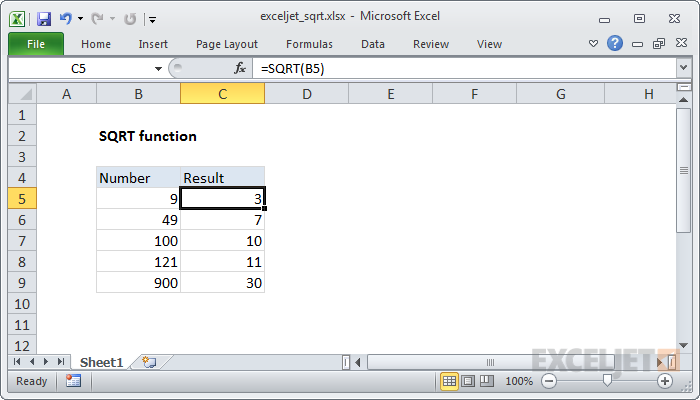# How to write in Radical Excel (second root)? (Step by step)

The second root of a number is a value that, when multiplied by itself, gives the number. The SQRT function in Excel returns the second root of a number. 1. First, to get the square root…